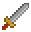# Math

## Vectors

Excalibur uses the Vector structure to represent points. The Vector class has many different static methods available for doing vector math as well as instance methods to combine vectors together in different ways.

### Creating vectors

To quickly create a vector, use the vec global:

``````import { vec } from 'excalibur'

const point = vec(0, 10)``````

Alternatively, you may see examples of using the more verbose `new Vector(x, y)` format:

``````import { Vector } from 'excalibur'

const point = new Vector(0, 10)``````

To set the value of an existing vector, use Vector.setTo:

``````import { vec } from 'excalibur'

const point = vec(0, 10).setTo(10, 10)``````

There are some built-in vector constants you can use:

### Cloning vectors

Vectors are objects, so mutating them will change the state for all references. Use the Vector.clone method to clone a vector to mutate it:

``````import { vec } from 'excalibur'

const point = vec(0, 10)
const samePoint = point.setTo(8, 8)
const anotherPoint = point.clone().setTo(50, 50)

console.log(point.toString()) // "(8, 8)"
console.log(samePoint.toString()) // "(8, 8)"
console.log(anotherPoint.toString()) // "(50, 50)"``````

Notice how both `point` and `samePoint` share the same vector reference, so using `setTo` mutates the vector. Use `clone` to ensure you are not changing vectors unexpectedly!

## Random

You can instantiate the Random class with an optional seed number. You can reuse this seed anytime to get the exact sequence of random numbers back. If no seed is provided, it uses `Date.now()` ticks as the seed.

``````const rand = new ex.Random(1234)

// random integer between [min, max]
rand.integer(0, 10)

// random floating number between [min, max)
rand.floating(0, 10)

// random true or false
rand.bool()
// random true or false with 40% likelihood of being true
rand.bool(0.4)

// next floating point between [0, 1)
rand.next()

// next integer between 0 and Number.MAX_SAFE_INTEGER
rand.nextInt()

// pick a random element from an array
rand.pickOne([0, 1, 4, 10])

// pick a 2 random elements from an array
rand.pickSet([0, 1, 4, 10], 2)
// pick a 4 random elements from an array, allowing duplicates
rand.pickSet([0, 1, 4, 10], 4, true)

// generate an array of 10 random numbers between [min, max]
rand.range(10, 0, 10)

// randomly shuffle an array using Fisher/Yates algorithm
rand.shuffle([0, 1, 2, 3, 4])

// Multi-sided dice helpers
rand.d4()
rand.d6()
rand.d8()
rand.d10()
rand.d12()
rand.d20()``````

A seeded random is very useful in games to do things like terrain generation, procedural content generation, etc. It allows you easily debug your algorithms by reusing the same seed, as well as to ensure your algorithms are deterministic.

##### Get Involved